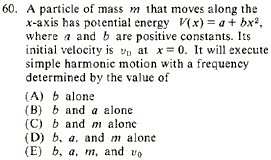GR 8677927796770177 | # Login | Register

GR8677 #60
Problem
 GREPhysics.NET Official Solution Alternate SolutionsMechanics}Simple Harmonic Motion

Recall . Simple harmonic motion has the simple form, . Thus , where is the frequency squared. Thus, simple harmonic motion occurs with a frequency determined by both and . This is choice (C).Alternate Solutions
 There are no Alternate Solutions for this problem. Be the first to post one!
ernest21
2019-08-23 02:03:37
I enjoyed reading your Solutions together with the answers of others. This is truly a great read for me. brick breaker 2a62
2016-09-20 02:36:59
Easy, but even easier if you look at it this way: a is absolutely meaningless because the zero of potential is arbitrary. So it\'s exactly like the mass-on-a-spring model.Almno10
2010-11-11 23:02:29
Force is the negative gradient of potential energy. The a drops out.
 psychonautQQ2013-09-26 08:41:23 but this is asking for the frequency, how does force and frequency relate?sirius
2008-11-05 22:26:45
A similar solution if you remember . The given V(x) has a vertical shift, a, which can be ignored by shifting your zero-point energy. So, , solving for makes it depend on b and m.

This is enough, but the problem asks for frequency . So . making f depend on b and m. The answer then is (C).
 alemsalem2010-09-21 06:25:37 i totally agree, to be sure that it doesn't depend on a, just remember that shifting the potential energy by a constant cannot change the motion (classically) so it doesn't affect the frequency.LaTeX syntax supported through dollar sign wrappers $, ex.,$\alpha^2_0$produces . type this... to get...$\int_0^\infty\partial\Rightarrow\ddot{x},\dot{x}\sqrt{z}\langle my \rangle\left( abacadabra \right)_{me}\vec{E}\frac{a}{b}\$